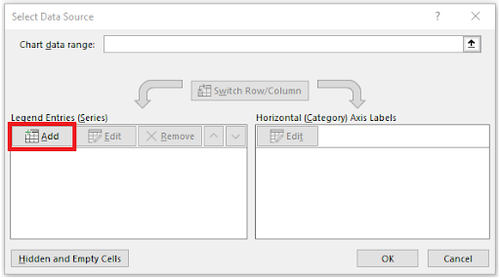# Difference between revisions of "Introduction to Microsoft Office"

Important: Please note that you must register on the EG1003 website before performing the lab.

# Objective

The objective of this lab is to use Microsoft Excel to analyze two case studies. The first case study will evaluate the acceleration due to gravity of a free-falling body and the second case study will analyze the historical trends for energy consumption in the United States.

# Overview

This lab is designed to teach the skills needed to become proficient with the three primary Microsoft applications: Excel, PowerPoint, and Word. These programs are widely used in academia and the public and private sectors so proficiency in these applications is a requirement. Completing assignments in EG1003 requires a basic competency in them and the following exercises are designed to create that competency.

## Microsoft Office

Microsoft Office is a software suite that bundles Microsoft Excel, PowerPoint, Word, and more.

Microsoft Excel is a spreadsheet program. Its function is collecting, processing, and analyzing data. Microsoft Excel has many features that streamline data analysis. It can be used to generate tables and graphs. It is useful for showing the relationships between data sets and identifying trends. Graphs will be required for many lab reports and presentations.

Microsoft PowerPoint is a presentation application. Its function is to create slides for presentations. In this course, eight lab presentations, three Milestone presentations for the semester long design project, and a final sales presentation for the semester long design project will be created and presented using PowerPoint.

Microsoft Word is a word processing application. Its function is writing and editing text. In this course, Microsoft Word will be used to write nine lab reports.

## Saving Work

The information on the Lab PCs cannot be accessed outside of the lab rooms. Email copies of all the files created to a personal account.

# Materials and Equipment

• A lab PC
• Microsoft Office suite

# Procedure

The templates created in this exercise for Microsoft Excel do not need to be submitted on the EG1003 website.

## 1. Microsoft Excel

In this exercise, common Excel equations and tools will be explored through the context of a problem.

A ball is dropped from the roof of the Bern Dibner Library building and the distance between the ball and the floor is measured and recorded as a function of time with a Lidar scanner. The time and distance data are shown in Table 1. The velocity, average velocity, and acceleration due to gravity of the ball will be calculated using Excel.

Table 1: Time and Height Data
Time (s) Distance (m)
0
19.620
0.2
19.424
0.4
18.835
0.6
17.854
0.8
16.481
1.0
14.715
1.2
12.557
1.4
10.006
1.6
7.063
1.8
3.728
2.0
0
1. Open the Microsoft Excel Template containing the above table.
2. The velocity of the ball is the change in distance over time. To calculate the instantaneous velocity at each point in time, a formula (1) is used.(1)

1. In (1),v is the instantaneous velocity,is the second distance point,is the first distance point,is the time corresponding to the second distance point, andis the time corresponding to the first distance point.
1. Assuming the initial velocity is zero, enter 0 into cell C2. In cell C3, enter the formula =(B3 - B2)/(A3 - A2). The velocity will be negative because the ball is traveling downwards.
3. Reselect cell C3. Click and drag from the bottom-right corner of the cell down to cell C12. This will copy the formula for the other cells in the column using the data in column A and column B (A3, A4, B3, B4 for cell C4, and so on).
4. To calculate the average velocity of the ball across the 12 data points, select cell D2. Enter the formula =AVERAGE(C2:C12).
5. Microsoft Excel is commonly used to visually represent data in graphs. Create a scatter plot for the relationship between velocity and time.
1. On the Excel ribbon, click on the Insert tab, and click Scatter on the Scatter option under the Charts group. This should create a blank graph, as shown in Figure 1.
File:Lab ScatterPlots Office.png
Figure 1: Inserting a Scatter Plot
2. Right-click on the empty graph and select Select Data.
3. Under the "Legends Entries(Series)" tab, click on the Add option, as shown in Figure 2. A new window called "Edit Series" will appear.Figure 2: Utilizing the Select Data Source Window
4. In the "Series X" values textbox, delete the {1} and highlight cells A2 to A12. In the "Series Y" values textbox, delete the {1} and highlight cells C2 to C12. Click "OK" to generate the scatter plot.
5. Add axis titles to the graph by clicking "Axis Titles" in the dropdown menu at the top right of the graph. Rename the axis and title.
6. Acceleration is the rate of change of the velocity, or the slope of the velocity vs. time graph. For roughly linear data on Microsoft Excel, a line of best fit is used to approximate the relationship between the data points in y = mx + b.
1. In the dropdown menu at the top right of the graph, hover over “Trendline” and click on the black arrow. Select "More Options".
2. Under the "Format Trendline" window, check off "Display Equation" on chart.
3. Check the slope of the graph and compare it to the acceleration due to gravity in New York, -9.802. Why is there a discrepancy?

# Assignment

The Excel file must be submitted to the EG1003 website by 11:59 PM the night before your next lab. There is no individual lab report or team presentation for this part of Lab 1.